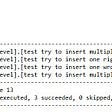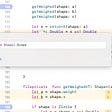Establish a pairs trading strategy between Evergreen Shipping and Yang Ming Shipping with Python.

# Highlights

• Difficulty:★★★☆☆
• Reminder: Pairs trading combines two or three types of assets with a hedging effect to eliminate market risks, obtain a market-neutral impact, and set entry and exit conditions to generate entry and exit signals in the asset price difference sequence. The concept of stationary state has been introduced before; this article further applies the theory of time series to pairs trading. If you want to know more theoretical derivation, you can refer to the actual practice of finance.

# Preface

When the market capital is excessively flooded, to avoid systemic risks, investors often establish long and short positions at the same time through asset allocation to eliminate most market risks and obtain stable returns. However, we select Evergreen and Yangming as the stock pair for pairs trading and use a single root test to determine whether the two-level spread has a stationary state. That is, it confirms that Evergreen and Yangming have a cointegration relationship. When the spread deviates, buy undervalued stocks, sell overvalued stocks, and reverse the position to earn the spread when the spread corrects.

# The Editing Environment and Modules Required

Windows OS and Jupyter Notebook

`# 基本功能import pandas as pdimport numpy as npfrom arch.unitroot import ADFimport statsmodels.api as sm# 繪圖import matplotlib.pyplot as pltplt.rcParams['font.sans-serif'] = ['Microsoft JhengHei']plt.rcParams['axes.unicode_minus'] = False# TEJ APIimport tejapitejapi.ApiConfig.api_key = 'Your Key'`

# Database Used

Return Information Data Table： Listed securities with the daily return. Code is ‘TWN/EWPRCD2’.

# Data Processing

Import the share price returns of Evergreen and Yang Ming from the TEJ database.

`# 匯入資料stock = tejapi.get('TWN/EWPRCD2',                   coid = ['2603','2609'],                   mdate= {'gte': '2019-06-01','lte':'2021-06-30'},                   opts={'columns':['coid','mdate','roia']},                   chinese_column_name=True,paginate=True)stock = stock.pivot(index='日期', columns='證券碼', values='日報酬率(%)')stock.columns = ['2603 長榮','2609 陽明']stock = stock * 0.01stock.tail(6)`

# Cointegration test

Step 1. The series of daily stock returns have a stationary state

We can see that the two-level daily rate of return fluctuates around 0 from the figure below, and we can confirm that the two-level daily rate of return series has a steady state.

`# 長榮與陽明 日報酬率的時序圖fig = plt.figure(figsize = (15,8))ax = fig.add_subplot()ax.plot(stock['2603 長榮'] ,linewidth=2, alpha=1)ax.plot(stock['2609 陽明'] ,linewidth=2, alpha=0.7)ax.axhline(0,color = 'black')ax.set_title('長榮與陽明 日報酬率的時序圖' ,fontsize=20 ,fontweight='bold')ax.legend(['2603 長榮','2609 陽明'],loc='best')ax.set_ylabel('報酬率', fontsize=12,rotation=0)ax.grid(axis='y')`

Step 2. Calculate the spread of the stock pair

First, We divide the backtest period into the formation and trading periods. When we calculate the spread sequence of the trading period to avoid the forward-looking bias, we use the alpha coefficient value and the beta coefficient value obtained from the linear regression of the spread series in the formation period to calculate the spread series for the trading period.

`# 價差def CointegrationSpread(df,formStart,formEnd,tradeStart,tradeEnd):    formX = df[(df.index >= formStart) & (df.index <= formEnd)]['2603 長榮']    formY = df[(df.index >= formStart) & (df.index <= formEnd)]['2609 陽明']    tradeX = df[(df.index >= tradeStart) & (df.index <= tradeEnd)]['2603 長榮']    tradeY = df[(df.index >= tradeStart) & (df.index <= tradeEnd)]['2609 陽明']        results = sm.OLS(formY,sm.add_constant(formX)).fit()    spread = tradeY - results.params - results.params * tradeX    return spreadSpread_2020_10_12 = CointegrationSpread(stock,'2019-06-01','2020-06-30','2020-10-01','2020-12-31')Spread_2021_01_03 = CointegrationSpread(stock,'2020-01-01','2020-12-31','2021-01-01','2021-03-30')# 對兩檔股價的價差序列做定態性檢定adfSpread = ADF(Spread_2021_10_12, trend='n')print(adfSpread.summary().as_text())`

We see in the below figure that we can reject the null hypothesis at the 1% significance level, indicating that the 2021_10_12 spread series is stationary. The daily return series of Evergreen and Yangming have a cointegration relationship.

We formulate trading strategies based on the opening and closing points,

• When the spread crosses μ+1.5σ, short the paired stocks and open a position in the opposite direction (sell Yangming; buy Evergreen).
• When the spread crosses below μ+0.2σ, long for paired stocks and close the position in the opposite direction.
• When the spread crosses μ−1.5σ, go long for paired stocks and open positions opposite (buy Yangming; sell Evergreen).
• When the spread crosses μ−0.2σ, short the paired stock and closes the position in the opposite direction.
• When the spread exceeds μ±2.5σ, the position will be closed immediately.
`Spread_2021_01_03 = Spread_2021_01_03.to_frame()Spread_2021_01_03.columns = ['價差']Spread_2021_01_03['開倉平倉區間'] = \    pd.cut(Spread_2021_01_03['價差'] ,           (float('-inf') ,mu-2.5*sd ,mu-1.5*sd ,mu-0.2*sd ,            mu+0.2*sd ,mu+1.5*sd ,mu+2.5*sd ,float('inf')) ,labels=False)-3Spread_2021_01_03['交易訊號'] = \    np.select([(Spread_2021_01_03['開倉平倉區間'].shift() == 1) &               (Spread_2021_01_03['開倉平倉區間'] == 2),                              (Spread_2021_01_03['開倉平倉區間'].shift() == 1) &               (Spread_2021_01_03['開倉平倉區間'] == 0),                              (Spread_2021_01_03['開倉平倉區間'].shift() == 2) &               (Spread_2021_01_03['開倉平倉區間'] == 3),                              (Spread_2021_01_03['開倉平倉區間'].shift() == -1) &               (Spread_2021_01_03['開倉平倉區間'] == -2),                              (Spread_2021_01_03['開倉平倉區間'].shift() == -1) &               (Spread_2021_01_03['開倉平倉區間'] == 0),                              (Spread_2021_01_03['開倉平倉區間'].shift() == -2) &               (Spread_2021_01_03['開倉平倉區間'] == -3)],                            [-2,2,3,1,-1,-3],default = 0)position = [Spread_2021_01_03['交易訊號']]ns = len(Spread_2021_01_03['交易訊號'])Spread_2021_01_03['倉位情況'] = pd.Series(position,index=Spread_2021_01_03.index)Spread_2021_01_03['倉位情況'] = Spread_2021_01_03['倉位情況'].shift() # 隔天開盤才進場Spread_2021_01_03 = Spread_2021_01_03.join(stock)Spread_2021_01_03['策略報酬率'] = \    np.select([Spread_2021_01_03['倉位情況'] == 1,               Spread_2021_01_03['倉位情況'] == 0,               Spread_2021_01_03['倉位情況'] == -1],              [Spread_2021_01_03['2609 陽明'] * -1 + Spread_2021_01_03['2603 長榮'] * 1,               0,               Spread_2021_01_03['2609 陽明'] * 1 + Spread_2021_01_03['2603 長榮'] * -1], default=np.nan)Spread_2021_01_03['累積報酬率'] = (Spread_2021_01_03['策略報酬率'] + 1).cumprod() -1Spread_2021_01_03.head(10)`

We complete the above strategy and present the strategy’s cumulative return and a maximum drawdown in the graph below.

# Conclusion

From the maximum drawdown chart, We can find that the maximum drawdown of the two pairs trade has fallen below 8% in a row, which means that the strategy’s stop-loss mechanism is not very good, or the μ mean and σ parameters are out of order. In the future, you can try to lower the standard of 2.5σ or calculate the μ mean and σ standard deviation of the formation period spread series in a rolling method because the volatility of maritime stocks in the first half of 2021 is extremely high. As a result, the trading period fell in 2021. From January to March 2020, the formation period is from January to December 2020.

The content of this webpage is not an investment device and does not constitute an offer or solicitation to offer or recommendation of any investment product. It is for learning purposes only and does not consider your individual needs, investment objectives, and specific financial circumstances. Investment involves risk. Past performance is not indicative of future performance. Readers are requested to use their independent thinking skills to make investment decisions independently. The author will not be involved if losses are incurred due to relevant suggestions.

# Source Code

【Data Analysis(10)】ARIMA-GARCH Model(Part 1)

You could encourage us by …
We will share financial database applications every week.
If you think today’s article is good, you can click on the
applause icon once.
If you think it is awesome, you can hold the
applause icon 50 times.

--

--

--

## The Launching of Raikoh Apps (Website)## 5 Secure Coding Tips in Go## Unit testing: How to write your first unit test for T-SQL code## Opaque Return Types Part 2## Has Azure Chargeback Improved with the New Cost Allocation Capabilities?## Rendering dunes terrain in WebGL## Terraform First Practice in Windows## 台灣經濟新報(TEJ)

TEJ為台灣本土第一大財金資訊公司，成立於 1990 年，專門提供金融市場基本分析所需資訊，以及信用風險、市場風險、資產評價、投資分析及公司治理分析等解決方案及顧問服務。鑒於財務金融領域日趨多元與複雜，TEJ 結合實務與學術界的精英人才，致力於開發機器學習ML、人工智慧AI及自然語言處理NLP等新技術，持續提供創新服務

## 【Quant(13)】RSI Indicator## Technical analysis of asset prices with Python## Thomas’ Proof of Value, January 2022## How To Write Custom Indicators in Python?